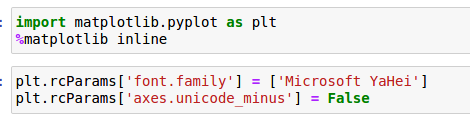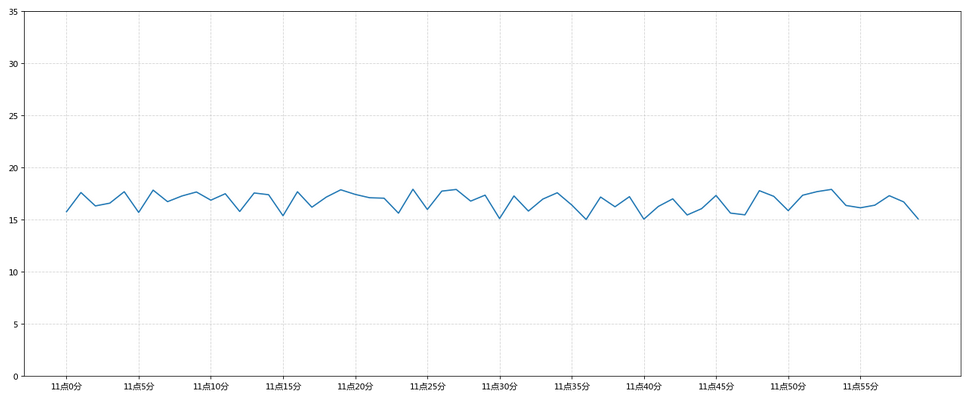Python的matplotlib包在设计之初并未考虑到中文字体显示的问题，因此需要自己将中文字体加入到matplotlib字体文件夹中，解决用matplotlib画图不显示中文字的问题。

## 问题出现

Python包matplotlib中文显示存在问题，如图：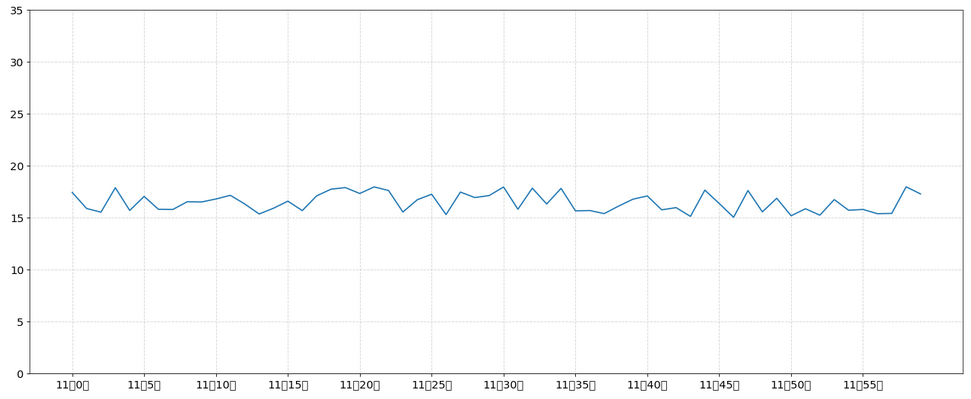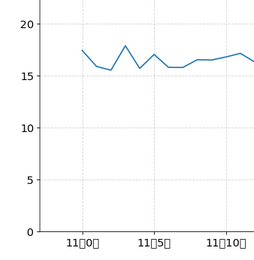## 解决步骤

### 2. 字体文件移入matplotlib字体文件夹

import matplotlib
print(matplotlib.__file__)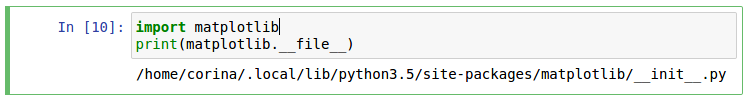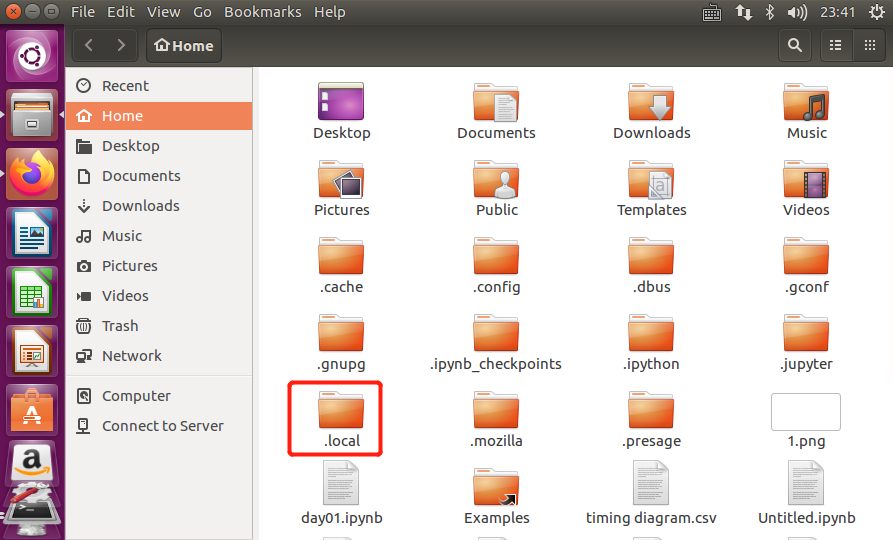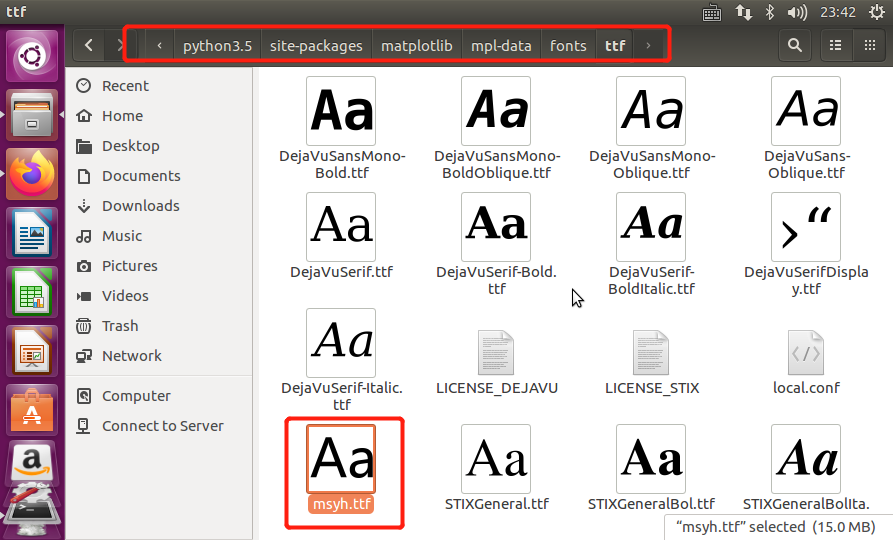### 3. 删除matplotlib缓存文件

import matplotlib
print(matplotlib.get_cachedir())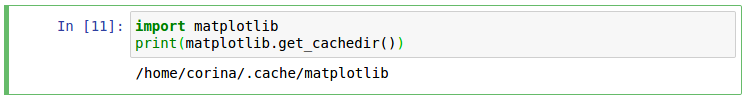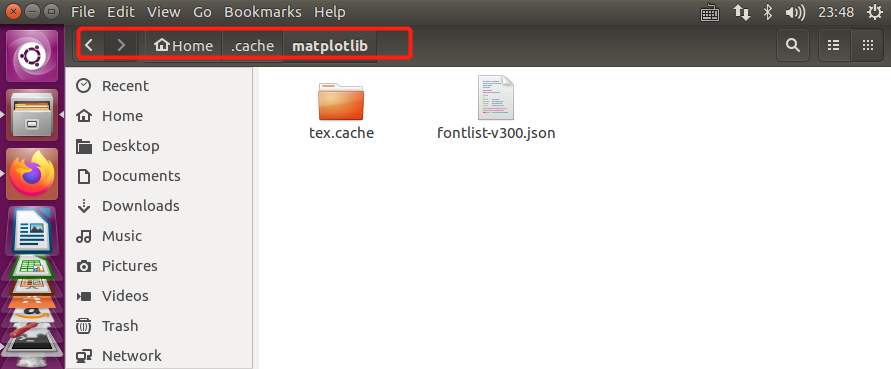### 5. 使用pyplot画图之前加入命令

plt.rcParams['font.family'] = ['Microsoft YaHei']
plt.rcParams['axes.unicode_minus'] = False